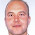# Learning Clojure

## Wednesday, March 10, 2010

### Moving Average of a List

Someone on Stack Overflow asked how to take the moving average of a list.

```;; The first one is elegant, but inefficient for long windows.
(defn average [lst] (/ (reduce + lst) (count lst)))
(defn moving-average [window lst] (map average (partition window 1 lst)))

;; The second is harder to understand, but keeps a running sum.
(defn partialsums [start lst]
(lazy-seq
(if-let [lst (seq lst)]
(cons start (partialsums (+ start (first lst)) (rest lst)))
(list start))))

(defn sliding-window-moving-average [window lst]
(map #(/ % window)
(let [start   (apply + (take window lst))
diffseq (map   - (drop window lst) lst)]
(partialsums start diffseq))))

;; Here is a list
(def integers (iterate inc 0))

;; Here are some averages of it:
(take 10 (moving-average 5 integers))
(take 10 (sliding-window-moving-average 5 integers))

```

Athena sprang fully-armed from the forehead of Zeus, and I have presented these functions in a similar spirit.

If anyone is interested in the gory details, I have a long file of increasingly pretty attempts, stack blowing versions, tail recursive versions, timings etc, which could be turned into a post if there is demand.

These two are not necessarily the fastest versions, but they are certainly the most elegant of the various versions that I tried, and fairly careful benchmarking has not shown any of my other versions to be consistently faster.

With the algorithms done, the next step would be to nail down the types and shell out to Java.

1.Would you be willing to contribute this to incanter.stats?

2.More than willing. Please take it and do as you will with it.

3.I realise I'm a bit late to the party but we came across this page looking for a quick solution - which worked fine. Now I had a bit of time to think about it - what do you think about the following implementation?
It's also completely lazy and I didn't notice any performance hits in a few quick unscientific tests I did. (sorry couldn't find a way of preformatting the code in the comment)

(defn sliding-window-moving-average [window lst]
(let [shiftedlists (take window (iterate (partial drop 1) lst))
window-sums (apply map + shiftedlists)]
(map #(/ % window) window-sums)))